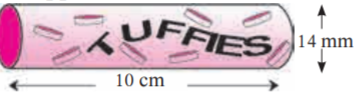# In the figure 7.12, a cylindrical wrapper of flat tablets is shown. The radius of a tablet is 7 mm and its thickness is 5 mm.

In the figure 7.12, a cylindrical wrapper of flat tablets is shown. The radius of a tablet is 7 mm and its thickness is 5 mm. How many such tablets are wrapped in the wrapper ?verified
Radius of a tablet, r = 7 mm
Thickness of a tablet, h = 5 mm
Radius of cylindrical wrapper, R =

$$\frac{14}{2}$$ = 7 mm
Height of wrapper (H) = 10 cm
= 10 × 10 mm
= 100 mm
Let n be the number of tablets wrapped in the wrapper.

$$n = \frac{\text{ Volume of the cylindrical wrapper} }{\text{ Volume of each tablet } }$$

$$\Rightarrow n = \frac{\pi R^2 H}{\pi r^2 h}$$

$$\Rightarrow n = \frac{\left( 7 \right)^2 \times 100}{\left( 7 \right)^2 \times 5} = 20$$

Thus, 20 tablets are wrapped in the wrapper.